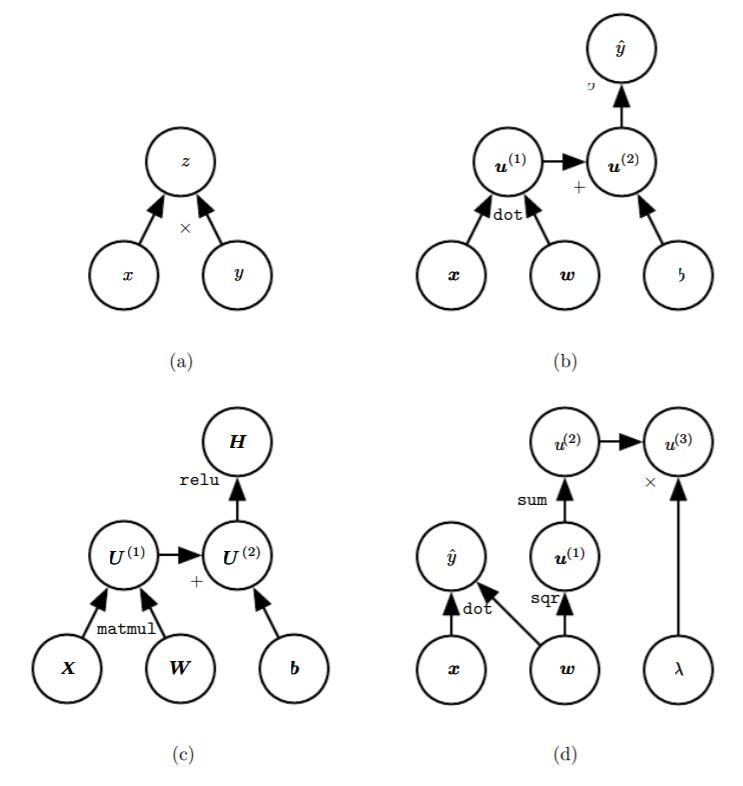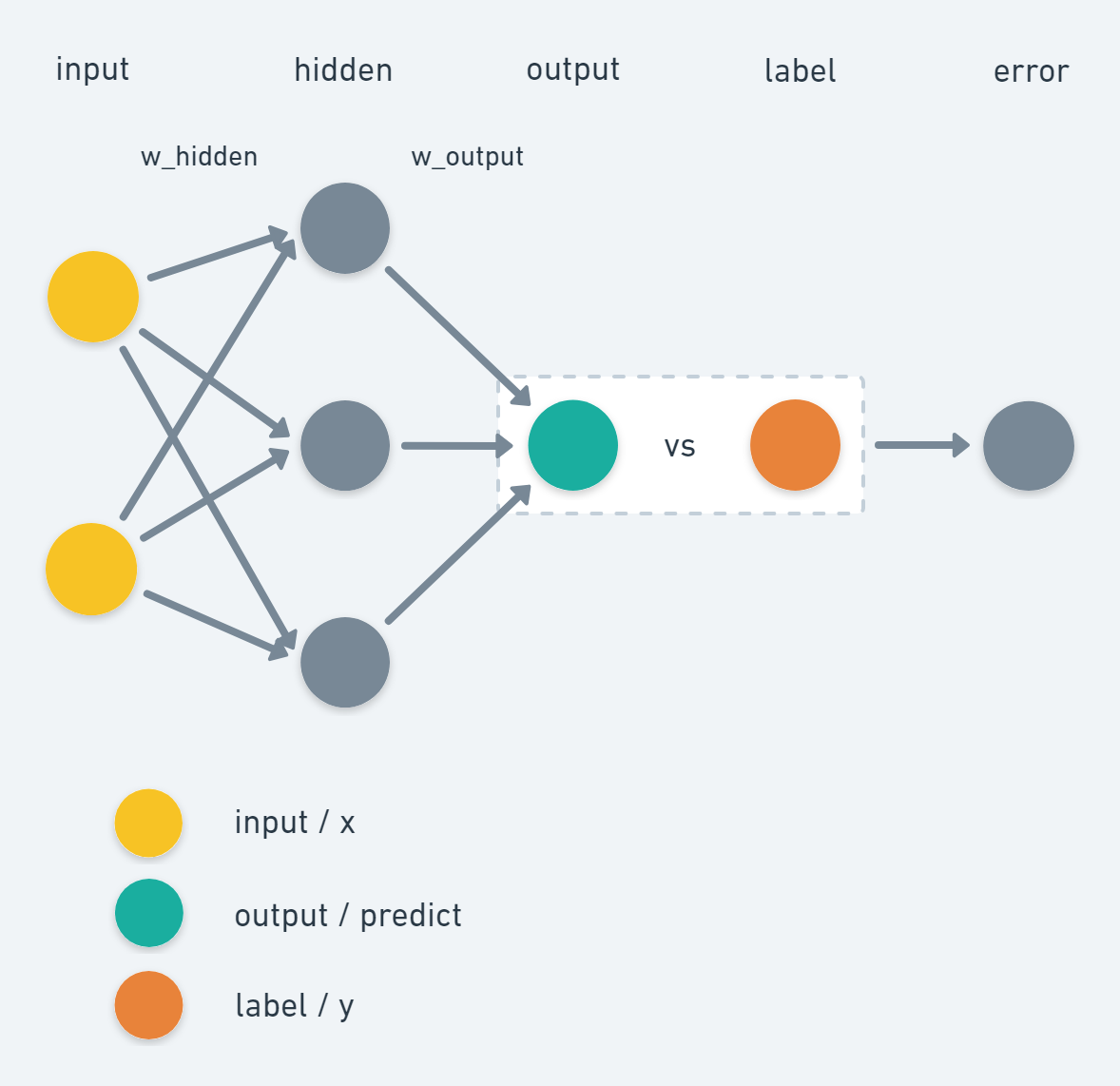### Deep Learning - Backpropagation

Wang Tiven September 04, 2018
425 favorite favorites
bookmark bookmark
share share

## 计算图图. 一些计算图的示例。(a) 使用 $$\times$$ 操作计算 $$z=xy$$ 的图。(b) 用于逻辑回归预测 $$\widehat{y}=\sigma(\boldsymbol{x}^\top \boldsymbol{y}+b)$$ 的图。一些中间表达式在代数表达式中没有名称，但在图形纸中却需要。我们简单地将第 i 个这样的变量命名为 $$\boldsymbol{u}^{(i)}$$ 。(c) 表达式 $$\boldsymbol{H}=\max\{0,\boldsymbol{X}\boldsymbol{W}+b\}$$ 的计算图，在给定包含小批量输入数据的设计矩阵 $$\boldsymbol{X}$$ 时, 它计算整流线性单元激活的设计矩阵 $$\boldsymbol{H}$$ 。(d) 示例 (a) 到 (c) 对每个变量最多只实施一个操作，但是对变量实施多个操作也是可能的。这里我们展示一个计算图，它对线性回归模型的权重 $$\boldsymbol{w}$$ 实施多个操作。这个权重不仅用于测试 $$\widehat{y}$$，也用于权重衰减罚项 $$\lambda\sum_iw_i^2$$

## 微积分中的链式法则

$\frac{dz}{dx}=\frac{dz}{dy}\frac{dy}{dx}$

$\displaystyle \frac{\partial z}{\partial x_i}=\sum_j\frac{\partial z}{\partial y_j}\frac{\partial y_j}{\partial x_i}$

$\displaystyle\nabla_\boldsymbol{x}z=\left(\frac{\partial \boldsymbol{y}}{\partial \boldsymbol{x}}\right)^\top\nabla_\boldsymbol{y}z$

Calculus on Computational Graphs: Backpropagation

Backpropagation

http://www.deepideas.net/deep-learning-from-scratch-i-computational-graphs/

## 多层感知器的反向传播算法

https://blog.csdn.net/qq_32611933/article/details/51612102

https://blog.csdn.net/zhuimeng999/article/details/80795943

$\displaystyle \mathcal{E}(n) = \frac 1 2 \sum_{j \in C} e_{j}^2(n)$

$\displaystyle \mathcal{E}_{av}(N) = \frac 1 {N} \sum_{n=1}^N \mathcal{E}(n) = \frac 1 {2N} \sum_{n=1}^N \sum_{j \in C} e_{j}^2(n)$

$e_j(n)=d_j(n)-y_j(n)$

$$\mathcal{E}(n)$$ 也就是第 n 个样本输入产生的输出层所有的输出的误差的平方和再除以 2 。除以 2 是为了后边求导时可以直接消掉。它也被称为全部瞬时误差能量

## 反向传播算法的实现

### 纯 Python 实现

https://medium.com/@curiousily/tensorflow-for-hackers-part-iv-neural-network-from-scratch-1a4f504dfa8# parameters and hyperparameters
epochs = 50000
input_size, hidden_size, output_size = 2, 3, 1
LR = .1 # learning rate


# Our data: x,y
X = np.array([[0,0], [0,1], [1,0], [1,1]])
y = np.array([ ,   ,   ,   ])


# Initlize wights
w_hidden = np.random.uniform(size=(input_size, hidden_size))
w_output = np.random.uniform(size=(hidden_size, output_size))


# Training
for epoch in range(epochs):

# Forward
act_hidden = sigmoid(np.dot(X, w_hidden))
output = np.dot(act_hidden, w_output)

# Calculate error
error = y - output

if epoch % 5000 == 0:
print(f'error sum {sum(error)}')

# Backward
dZ = error * LR
w_output += act_hidden.T.dot(dZ)
dH = dZ.dot(w_output.T) * sigmoid_prime(act_hidden)
w_hidden += X.T.dot(dH)


def sigmoid(x):
return 1 / (1 + np.exp(-x))

def sigmoid_prime(x):
return x * (1 - x)


### TensorFlow 的实现

http://blog.aloni.org/posts/backprop-with-tensorflow/